What error rates can we expect for a trained classifier? How good or bad is a 50% error? Well, if classes are separable, a zero-error classifier is possible. But a very bad classifier may assign every object to the wrong class. Generally, all errors between zero and one are possible:.

Much more can be said for given class prior probabilities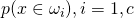, number of classes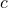and  class overlap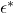.

#### The classification error and the Bayes error

Class overlap arises if objects belonging to different classes may have the same representation. Assume that the class probabilities or densities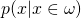as well as the class prior probabilities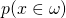(which is the probability that an arbitrary object belongs to class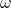), are known for all classes. Then we will assign every objectto the most probable class given. This is the class for which the posterior probabilityis maximum. Let this be class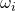. The error contribution of a vectoris the probability that the objectoriginates from another class  than this one.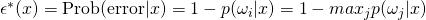The * indicates that this is the minimum error. The overall error for an arbitraryis therebywhich is the best possible result, the so called Bayes error. It can only be reached for known probability functionsand class priors. What happens if we don’t know them and sometimes assignto classfor which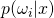is not the largest? Then the error will increase, so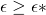. In the worst case everywill be assigned to the classfor which the posterior probabilityis minimum instead of maximum. So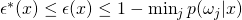For two-class problems it holds that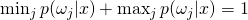as the sum of all probabilities for a givenshould be one and there are just these two. Consequently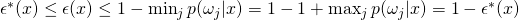by which we find, after taking the expectation that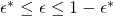for any classifier with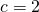However, forthe minimum posterior for every pointwill beas it is possible that other posteriors sum to one. So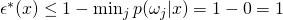and thereby we find for the multi-class casefor any classifier with#### Using a random, prior sensitive classifier

What will happen if we have chosen by accident a bad classifier, e.g. one that uses random assignment, or a classifier like the nearest neighbor rule while the representation is random? With a probability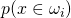an object of classwill be encountered and with a probabilityit will not be assigned to its own class but a wrong class. This results in an errorThis will be maximum in case ofequal probable classes.  They will all have a prior probability of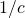by whichfor a random, prior sensitive classifier

#### Classification using class priors only

From the above it is clear that bad classifiers may yield large errors. The error of two-class classifiers is bounded by the class overlap. Multi-class classifiers are unbounded from above and may result into an error of one. If one has some doubts about the representation, the features or the classifier, e.g. after inspecting the results of some cross-validation experiments, a better result can be realized on the basis of the prior probabilities. If they are known (or if the estimate on the basis of the training set is reliable) then assigning all objects to the most probable class will classify all objects of this class correctly. All objects of the other classes will be classified wrongly, resulting in the following error: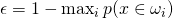for a classifier based on class priors only

For two-class classifiers with equally probable classes this results in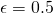. If the classes are not equally probable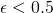. A 50% error is thereby the worst thing that may happen if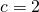and no proper representation is available.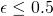for two-class problems and a classifier based on class priors only

#### How bad is 50% error for a multi-class problem?

A 50% error is the worst that should be found for two-class problems. Only in case of well-separable classes and the use of a very stupid classifier larger error rates may result. This can easily be avoided by assigning all objects to the most probable class. However, in case of multi-class problems much larger errors may be found. How good is a 50% error for a multi-class classifier? Can we compare it in one way or the other with a two-class performance?

To answer this question we have a look at the confusion matrix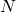resulting from a-class classifier. It is amatrix in which elementlists the number of objects with true classand that are assigned to class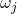. The numbers on the diagonal refer to correctly classified objects. The classification error is thereby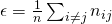Let the total number of objects be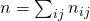. The error contribution of an arbitrary two class problem taken out of this multi-class problem is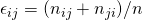. In general they will not be equal, but in order to get a feeling for the above question we will assume they are. As there are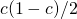two-class problems in a-class problem, the average contribution of a two-class confusion to the overall classification error is. In such a two-class problem just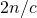objects are involved instead of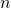. Its two-class error probability for theseobjects is thereby a factorlarger. Consequently, the average two-class error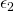relates to the-class erroras follows: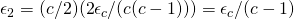To answer the question: a 50% error in a 50-class problem is about as good as a 1% error in a two-class problem, as every two-class problem in the 50-class problem has on the average to be solved with this performance to reach an overall error of 50%.

Filed under: ClassificationEvaluation